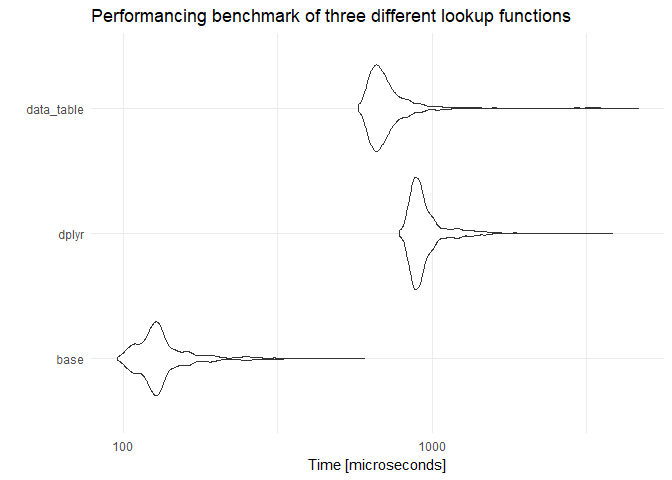# An R vlookup? Not so silly idea

[This article was first published on Digital Age Economist on Digital Age Economist, and kindly contributed to R-bloggers]. (You can report issue about the content on this page here)
Want to share your content on R-bloggers? click here if you have a blog, or here if you don't.

It started out as a joke, but Jenny Bryan recently posted a `vlookup` implementation in `R`. Here is the original post as seen on twitter:

The argument for the creation of this kind of lookup implementation in `R`, was to help facilitate a join that wasn’t covered by the standard joins. This tweet started out as a joke function that would surely never see the daylight of production code, but there was one thing that stood out about the small little function – it was written in `base` R and made use of R’s list data objects. What makes this intriguing you ask? Well, combining `lists` and `base` usually has a nice consequence – speed.

So that is what I set out to test: How much more efficient would this `vlookup` be when compared to a `dplyr` or `data.table` implementation? We start off by recreating the vlookup function and use the tweet’s example to show its use case:

``````library(tidyverse)

vlookup <- function(this, df, key, value) {
m <- match(this, df[[key]])
df[[value]][m]
}

vlookup_base <- function(){
c("Luke Skywalker", "Jabba Desilijic Tiure", "Yoda") %>%
vlookup(starwars, "name", "mass") %>%
sum
}
vlookup_base()
``````
``````##  1452
``````

Next we build the same query, but using `dplyr` notation to give us the same results (a quick check shows us the sum results are the same):

``````vlookup_dplyr <- function(){
starwars %>%
filter(name %in% c("Luke Skywalker", "Jabba Desilijic Tiure", "Yoda")) %>%
pull(mass) %>%
sum
}
vlookup_dplyr()
``````
``````##  1452
``````

Lastly we build the query using `data.table`. This implementation comes from the comment section of the tweet. We first have to coerce the `starwars` data into a data.table object for this to work. We also lose the `%>%` workflow which can be quite a pain. In this case it doesn’t matter, because we all about speed!

``````library(data.table)
vlookup_dt <- function(){
name_vec <- c("Luke Skywalker", "Jabba Desilijic Tiure", "Yoda")
df <- data.table(dplyr::starwars)
df[.(name_vec), on = 'name', sum(mass)]
}
``````

To test the speed to all of these function, we will use the `microbenchmark` library. Its one of my favourite libraries in `R`,thanks to its ease of use and quick API to comparing functions.

``````library(microbenchmark)
microbenchmark(
base = vlookup_base(),
dplyr = vlookup_dplyr(),
data_table = vlookup_dt(),
times = 1000L
)
``````
``````## Unit: microseconds
##        expr      min        lq      mean   median       uq       max neval
##        base   93.539  116.1030  143.8219  129.642  147.692  4797.951  1000
##       dplyr  780.309  889.0265 1017.7738  943.795 1035.898  8516.107  1000
##  data_table 1030.564 1166.3595 1454.0140 1252.103 1441.641 50518.175  1000
##  cld
##  a
##   b
##    c
``````

Here we see the enormous speed gain we got from using `vlookup_base` in comparison with the other two frameworks. In all fairness, I feel that I might be handicapping `data.table` a bit, just to the coercion of the `starwars` dataset each time. So lets see what happens when I do the coercion outside the function.

``````df <- data.table(dplyr::starwars)
vlookup_dt <- function(){
name_vec <- c("Luke Skywalker", "Jabba Desilijic Tiure", "Yoda")
df[.(name_vec), on = 'name', sum(mass)]
}
res_mic <- microbenchmark(
base = vlookup_base(),
dplyr = vlookup_dplyr(),
data_table = vlookup_dt(),
times = 1000L
)
res_mic
``````
``````## Unit: microseconds
##        expr     min      lq     mean  median       uq      max neval cld
##        base  95.590 118.975 137.5330 128.410 140.1030  603.077  1000 a
##       dplyr 784.410 873.026 962.0909 911.180 972.7185 3840.002  1000   c
##  data_table 579.693 648.206 740.3640 685.129 746.0520 4667.900  1000  b
``````

We definitely gained some speed by not having to coerce the data.frame over and over, but, the overall speed comparison is still nothing like the old fashion `vlookup_base`.R-bloggers.com offers daily e-mail updates about R news and tutorials about learning R and many other topics. Click here if you're looking to post or find an R/data-science job.
Want to share your content on R-bloggers? click here if you have a blog, or here if you don't.